Courses

# Test: Crystal Field Theory (CFT) And Colour Of Complexes

## 25 Questions MCQ Test Chemistry Class 12 | Test: Crystal Field Theory (CFT) And Colour Of Complexes

Description
This mock test of Test: Crystal Field Theory (CFT) And Colour Of Complexes for JEE helps you for every JEE entrance exam. This contains 25 Multiple Choice Questions for JEE Test: Crystal Field Theory (CFT) And Colour Of Complexes (mcq) to study with solutions a complete question bank. The solved questions answers in this Test: Crystal Field Theory (CFT) And Colour Of Complexes quiz give you a good mix of easy questions and tough questions. JEE students definitely take this Test: Crystal Field Theory (CFT) And Colour Of Complexes exercise for a better result in the exam. You can find other Test: Crystal Field Theory (CFT) And Colour Of Complexes extra questions, long questions & short questions for JEE on EduRev as well by searching above.
QUESTION: 1

### Direction (Q. Nos. 1-10) This section contains 10 multiple choice questions. Each question has four choices (a), (b), (c) and (d), out of which ONLY ONE is correct. Q.  Among the following complexes the one which shows zero crystal field stabilisation energy is

Solution:

In [Fe(H2O)6]3+, iron has 3d5 configuration. Since, H2O is weak ligand the distribution is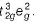.
Hence, CFSE = - 3 x 0.4 + 2 x 0.6 = 0.0

QUESTION: 2

### The wavelength of light absorbed is highest in

Solution:

Among all the coordination aforesaid in complex [Co(NH3)5Cl]2+ unpaired electrons are present with the central element cobalt so it required less energy for excitation. Thus, the wavlength of light absorbed will be the highest.

QUESTION: 3

### The CFSE for octahedral [CoCI6]4- is 18000 cm-1. Then the CFSE for tetrahedral [CoCI4]2- will be

Solution:

CFSE for [CoCI4]2- will be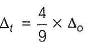i.e.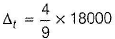= 8000cm-1

QUESTION: 4

The crystal field splitting energy (Δo) of

I. [CoBr6]3- II. [CoF6]3-
III. [Co(NCS)6]3- IV. [Co(CN)6]3- is in the order of

Solution:

Crystal field splitting energy depends on strength of ligand. Strong ligands have more value of CFSE (Δo).
Hence, Br- < F- < NCS < CN-

QUESTION: 5

A magnetic moment of 1.73 BM will be shown by one among the following

Solution:

The correct answer is Option A.
Electronic configuration of Cu2+  ion in [Cu(NH3)4]2+.
Cu2+ ion =[Ar]3d94s0.
∴Cu2+ ion has one unpaired electron.
Magnetic moment of [Cu(NH3)4]2+ (μ) =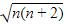BM
where, n = no. of unpaired electrons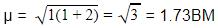Whereas Ni2+ in [Ni(CN)4]2− , Ti4+ in TiCl4 and Co2+ ion [COCl6]4− has 2,0 and 3 unpaired electrons respectively.

QUESTION: 6

The correct order o f ligand field strength is

Solution:
QUESTION: 7

The crystal field splitting energies (CFSE) of high spin and low spin d6 metal complexes in octahedral complex in terms of Δo respectively are

Solution:

For high spin d6, CFSE = - 4 x 0.4 + 2 x 0.6 = -0.4
For low spin d6, CFSE= - 6 x 0.4 = -2.4

QUESTION: 8

The Δt of the following complexes follows the order

I. [CoCI4]2-

II. [CoBr4]2-

III. [Co(NCS)4]2-

Solution:

Explanation : Bonding Energy :

[CoCI4]2      -2084.67

[CoBr4]2      -3139.39

[Co(NCS)4]2-  - 8559.48

Correct order is : III > II > I

QUESTION: 9

In which of the following coordination entities, the magnitude Δo (CFSE in octahedral field) will be maximum ?

Solution:

Increasing order of ligand field strength
l- < Br- < SCN- < CI- < S2- < F- < OH-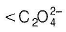< H2O < NCS- < edta4- < NH3 < en < CN- < CO

QUESTION: 10

The increasing order of wavelength of absorption for the complex ions

I. [Cr(NH3)6]3+

II. [CrCI6]3-

III. [Cr(H2O)6]3+
IV. [Cr(CN)6]3-

is :

Solution:

Stronger ligand causes greater splitting between two energy levels.

The energy of light absorbed is more and the wavelength of light absorbed is less for the stronger ligand.

Hence order of wavelength absorption will be reverse of the order of the strength of ligands.

Hence A is correct.

*Multiple options can be correct
QUESTION: 11

Direction (Q. Nos. 11-15) This section contains 5 multiple choice questions. Each question has four choices (a), (b), (c) and (d), out of which ONE or MORE THAN ONE are correct.

Q.

Which of the following metal ions cannot form both high spin and low spin octahedral complexes?

Solution:

Ti3+ has 3d1 configuration and Cu2+ has 3d9 configuration.

*Multiple options can be correct
QUESTION: 12

Which is/are the correct statements?

Solution:

If Δo < P high spin complexes are favoured.

*Multiple options can be correct
QUESTION: 13

CFSE is zero for the complexes with

Solution:

For d5 in weak field, CFSE = -3 x 0.4 + 2 x 0.6 = 0
For d10 in weak and strong ligand field, CFSE = -6 x 0.4 + 4 x 0.6 = 0

*Multiple options can be correct
QUESTION: 14

CFSE value depends on

Solution:

Geometry of complex, charge on metal ion, nature of ligands and series of d orbital are the factors on which CFSE value depends.

*Multiple options can be correct
QUESTION: 15

Octahedral complex of Cr (III) will be

Solution:

Cr (III) ion have 3d3 configuration.
Cr (III) ion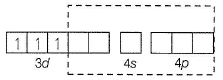Flence, it will form always d2sp3 hybridisation and show colour due to the presence of 3 unpaired electrons.

QUESTION: 16

Comprehension Type

Direction (Q. Nos. 16 and 17) This section contains a paragraph, describing theory, experiments, data, etc. Two questions related to the paragraph have.

Passage

In octahedral complexes due to repulsion between the ligands and d-orbitals, there is splitting of d-orbitals into two sets, i.e. two orbitals of higher energy called eg and three orbitals of lower energy called t2g. The difference of energy between the two sets of d-orbitals is called crystal field stabilisation energy denoted by Δo. For any given metal cation, the magnitude of Δo depends on the nature of ligands.

Q.

The CFSE for d7 configuration for strong ligand field is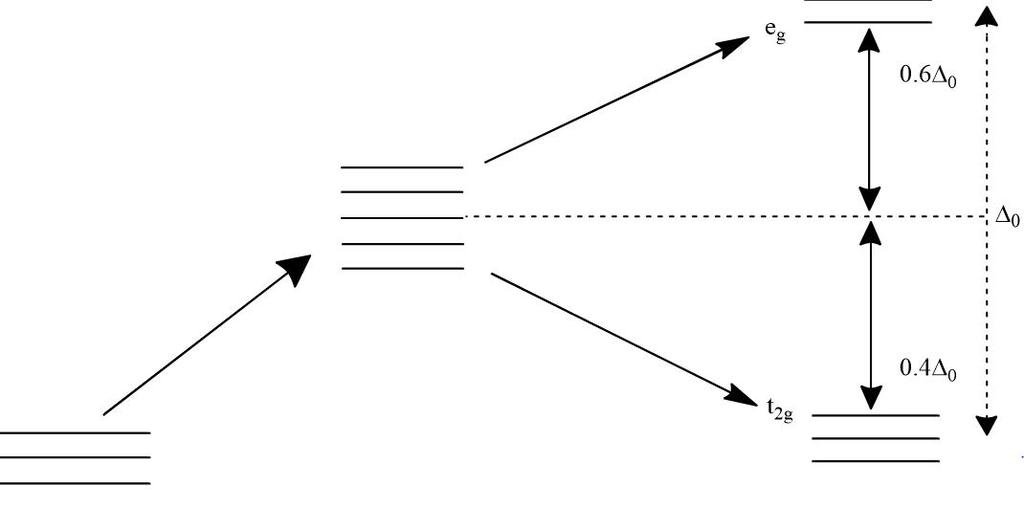Solution:

The total crystal field stabilisation energy is given by CFSE(octahedral) =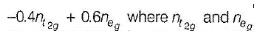are the number of electron occupying the t2g and eg orbitatls respectively.
For d7 in strong field,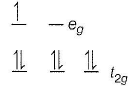CFSE = -0.4 x 6 + 0.6 x 1 = -2.4 + 0.6 = -1.8Δo

QUESTION: 17

In octahedral complexes due to repulsion between the ligands and d-orbitals, there is splitting of d-orbitals into two sets, i.e. two orbitals of higher energy called eg and three orbitals of lower energy called t2g. The difference of energy between the two sets of d-orbitals is called crystal field stabilisation energy denoted by Δo. For any given metal cation, the magnitude of Δo depends on the nature of ligands.

Q.

In the following complexes of manganese, the distribution of electrons in d-orbitals of manganese

i. [Mn(H2O)6]2+

ii. [Mn(CN)6]4-

Solution:

In [Mn (H2O)6]2+, manganese have d5 configuration. H2O is a weak field ligand. Hence, it will form high spin complex.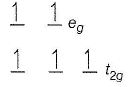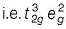In [Mn(CN)6]4-, manganese have d5 configuration. CN- ion is a strong field ligand. It will form low spin complex by pairing of electron in t2g orbitals.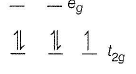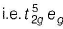QUESTION: 18

Matching List Type

Direction (Q. No. 18-19) Choices for the correct combination of elements from Column I and Column II are given as options (a), (b), (c) and (d) out of which one is correct.

Q.

Match the Column I with Column II and mark the correct option from the codes given below.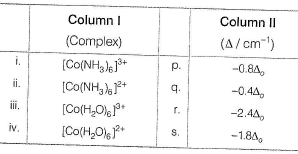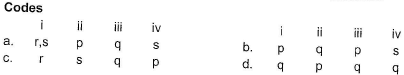Solution:

(i) → (r), (ii) → (s), (iii) → (q), (iv) → (p)

QUESTION: 19

Match the Column I with Column II and mark the correct option from the codes given below.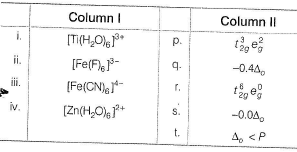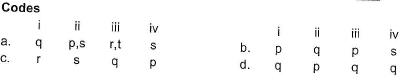Solution:

(i) → (q), (ii) → (p,s), (iii) → (r,t), (iv) → (s)

*Answer can only contain numeric values
QUESTION: 20

One Integer Value Correct Type

Direction (Q. Nos. 20-24) This section contains 5 questions. When worked out will result in an integer from 0 to 9 (both inclusive).

Q.

Number of unpaired electrons in d6, low spin octahedral complex.

Solution:

In d6 low spin octahedral complex.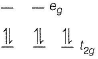Hence, zero number of unpaired electrons.

*Answer can only contain numeric values
QUESTION: 21

The number of ligands which have strong crystal field splitting than

H2O among SCN-, NCS-, EDTA4- ,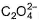,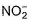, Br-, PPh3, F-

Solution:

NCS- edta4- ,and PPh3 are strong field ligand than H2O.

*Answer can only contain numeric values
QUESTION: 22

The total number of unpaired electrons in the two complexes [Cr(H2O)6]2+ and [Cr(CN)6]4- having octahedral geometry are

Solution:

In [Cr(H2O)6]2+ chromium have d4 configuration and H2O is a weak field ligand.
d4 (weak field)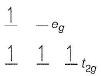In [Cr(CN)6]4- chromium have d4 configuration and CN- ion is a strong field ligand. Hence, CN- ion causing pairing of electron. d4 (strong field)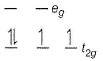Hence, total number of unpaired electrons in both the complex are 6.

*Answer can only contain numeric values
QUESTION: 23

Number of unpaired electrons in t2g and eg orbitals in weak octahedral ligand fields with d7 configuration.

Solution:

d7 (weak octahedral field)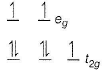Hence, 3 unpaired electrons.

*Answer can only contain numeric values
QUESTION: 24

In tetrahedral complexes having weak field ligand, pairing starts with ...... electron.

Solution:

The pairing starts with six electrons in the tetrahedral complexes which have weak field ligand.

QUESTION: 25

Statement Type

Direction (Q. No. 25) This section is based on Statement I and Statement II. Select the correct answer from the codes given below.

Q.

Statement I : The value of CFSE for the octahedral complex [Co(NH3)6]3+ is - 2.4Δo.

Statement II : The ammonia molecule is strong field ligand.

Solution:

In [Co(NH3)6]3+ Co, have d6 configuration and NH3 is strong field ligand.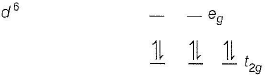CFSE(OCt) = -0.4 x 6 + 0.6 x 0 = -2.4Δo